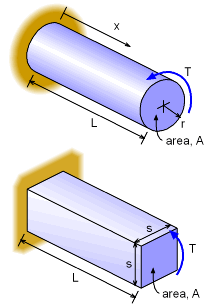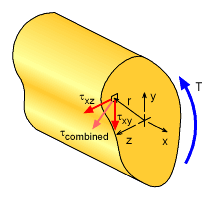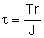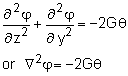Ch 2. Torsion Multimedia Engineering Mechanics CircularBars Nonuniform &Indeterminate Thin-walledTubes Non-Circular Bars
 Chapter 1. Stress/Strain 2. Torsion 3. Beam Shr/Moment 4. Beam Stresses 5. Beam Deflections 6. Beam-Advanced 7. Stress Analysis 8. Strain Analysis 9. Columns Appendix Basic Math Units Basic Equations Sections Material Properties Structural Shapes Beam Equations Search eBooks Dynamics Fluids Math Mechanics Statics Thermodynamics Author(s): Kurt Gramoll ©Kurt GramollMECHANICS - CASE STUDY IntroductionCircular and Square Bars Subjected to a Torque, T A new car engineer notices that solid square steel rods are less expensive per pound to purchase than solid circular steel rods. He thinks he can save the company money by specifying square rod for the drive shafts in all vehicles. What is known: Each rod has the same cross sectional area, A. The same torque, T, is applied to both rods. The material shear modulus is not given bit both rods are the same material. Question For the same cross sectional area and applied torque, what is the ratio of maximum shear stress in the square rod to the circular rod? ApproachShear Stress with Torque about the x-axis The maximum shear stress for the circular solid rod can be found using previously derived torsional shear stress equation for round bars,For the square solid rod, a new equation needs to be derived. The equation will be a form of the Poisson's equation,where φ is a scalar stress function such that     τxy = -dφ/dz     τxz = +dφ/dy The differential equation needs to be solved for a square boundary where φ is set to zero. This problem requires basic elasticity theory. An introduction will be presented for simple torque applied to a non-circular rod or shaft.

Practice Homework and Test problems now available in the 'Eng Mechanics' mobile app
Includes over 400 problems with complete detailed solutions.
Available now at the Google Play Store and Apple App Store.